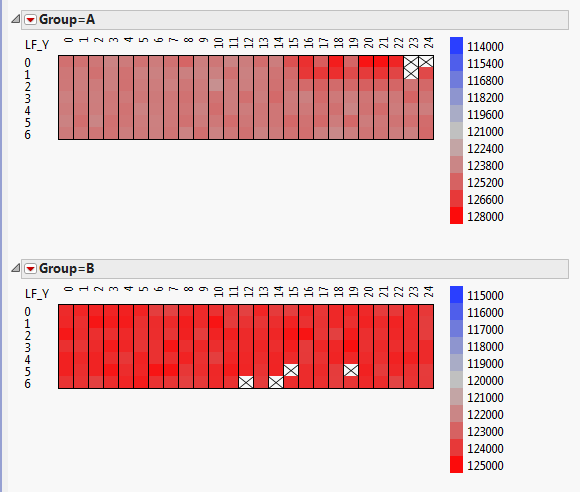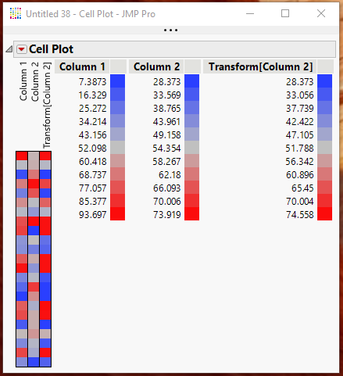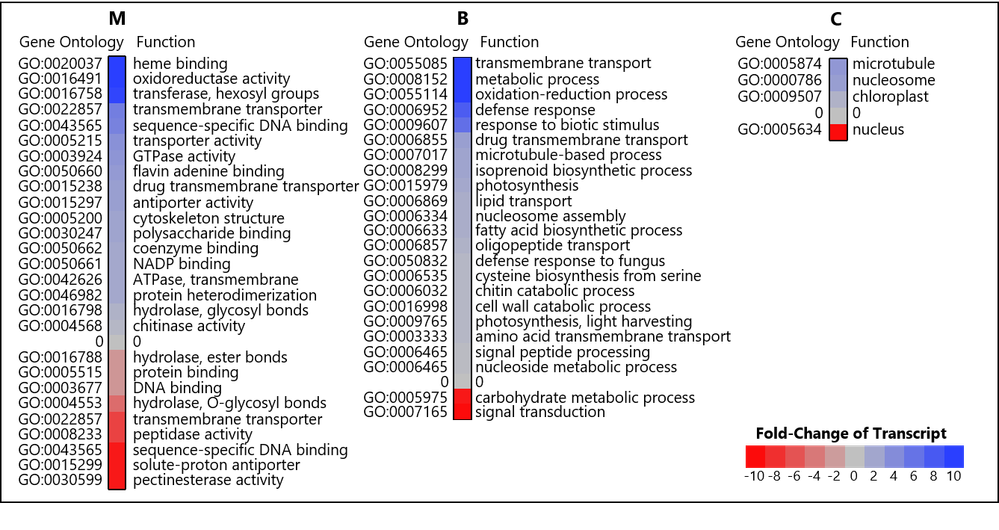Choose Language Hide Translation Bar
Highlighted

## How to set min/max colour gradient for cell plot

Hello,

I have cell plots from a table, in groups.

The cells are coloured by gradient according to the min/max in a group; each group has a different min/max.

Is it possible to set each group to have the same min/max colour gradient?4 REPLIES 4
Highlighted

## Re: How to set min/max colour gradient for cell plot

You should get the results you want if you select the "Use Uniform Scaling" on the initial dialog box.

You can also get what you want by changing the Modeling Type to Nominal, and then set the cell color in a script, based upon the scaling and coloring you want.
Jim
Highlighted

## Re: How to set min/max colour gradient for cell plot

Dear Jim,

This kind of plot would do the trick of multiple Y axes

However the scaling problem mentioned by charleslean is not easily overcome!

Is there a script I can use, because 1) uniform scaling does not work since it makes the range too wide and 2) I did not understand how to implement cell color according to nominal modeling.

Here is the cell plot script output from the dialog menu:

``````Cell Plot(
Scale Uniformly( 1 ), // Ideally there should be a way to set max and min range for the color plot here
Center at zero( 1 ),
Y( :Name( "% of transcript" ) ),
X( :Function Class ),
Label( :Gene Ontology, :Function ),
Legend( 1 ),
SendToReport(
Dispatch( {}, "Cell Plot Report", FrameBox, {Frame Size( 616, 106 )} ),
Dispatch( {}, "Cell Plot Report", FrameBox( 3 ), {Frame Size( 616, 106 )} ),
Dispatch( {}, "Cell Plot Report", FrameBox( 5 ), {Frame Size( 616, 106 )} ),
Dispatch( {}, "", NumberColBox, {Set Format( "Fixed Dec", 6, 0 )} )
)
);``````

Your wizardry would be greatly appreciated!

Best, ezra

Highlighted

## Re: How to set min/max colour gradient for cell plot

You can accomplish the graph colorization the way you want, by creating a transform column.  In the example below, the data table has Column 1 with a range of 7.3-93.9, while Column 2 has a range of 28.4-73.9.  As can be seen in the Cell plot, the color patterns are very different for ranges of Column 1 and Column 2.  However, when Column 2 is transformed, the color ranges are the same.The key is to create the transform column using the following formula

``````If(
:Column 1 < 25, x = Col Min( :Column 2 ),
:Column 1 > 75, x = Col Max( :Column 2 ),
x = :Column 1
);
x;``````

From a scripting standpoint, if you wanted to create a Cell Plot on Column 1 and Column 2, but restrict the color rage to the smaller range from Column 2, here is the script that would do that

``````Cell Plot(
Scale Uniformly( 0 ),
Center at zero( 0 ),
Y(
:Column 2,
Transform Column(
"Transform[Column 2]",
Formula(
If(
:Column 1 < 25, x = Col Min( :Column 2 ),
:Column 1 > 75, x = Col Max( :Column 2 ),
x = :Column 1
);
x;
)
)
),
Legend( 1 )
);``````
Jim
Highlighted

## Re: How to set min/max colour gradient for cell plot

Dear Jim,

As a matter of fact I did something very similar! I manually changed the extreme values to a max and min of 10 and -10, respectively. That way the legend is the same for all graphs. and I preserve sensitivity to changes that are close to the middle (0) Here is the result:This is a publication ready graph after much manipulation. I really wish there was more integration between the cell plot which creates nicely standardized color maps and graph builder functionality (e.g. legend labels etc)